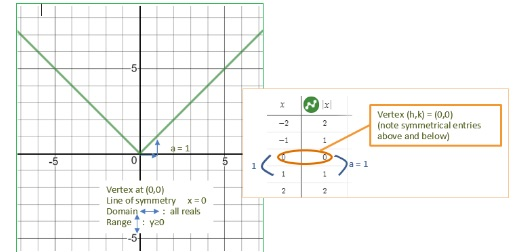# LINR 3|Lesson 3| Try This! Absolute Value Parent Function Solution

Vertex:  $$(0,0)$$

Axis of Symmetry:  $$x = 0$$

Increasing:  $$xge0$$;  Decreasing $$-∞< x<0$$

Domain:  $$-∞< x< ∞$$  Range:  $$yge0$$

Rewrite as a piecewise function:Lets take a closer look at parent functions: